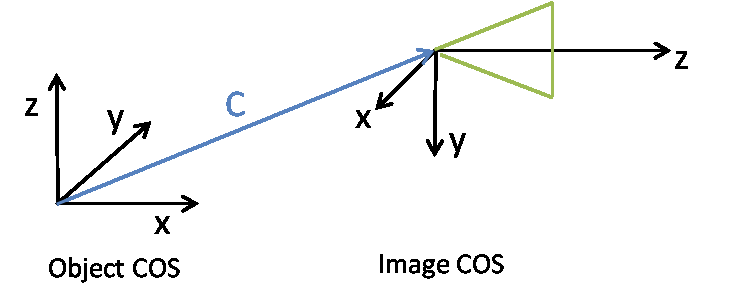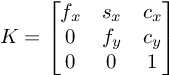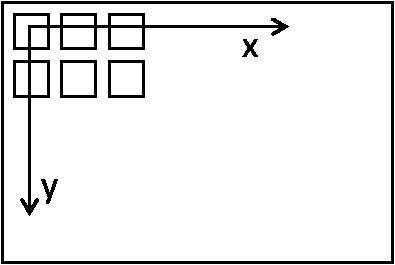### Coordinate Systems

The definitions and specifications of the different coordinate systems related to SURE are described below.

### Input/output Coordinate System

• SURE expects the camera orientation and/or LiDAR point clouds to be defined in the same Cartesian coordinate system. If your input data is defined in a projected coordinate system please see the article on Earth Curvature.

• You can specify the coordinate system of your data during the project set up or in the Advanced Configuration Panel. See Georeferencing and Tiling for details.

• All output results are generated in the same coordinate system as the input data except for Cesium and slpk mesh formats, which require a specific coordinate system. In this special cases, the project must use a coordinate system for which transformations are supported. To make it possible to generate these output formats based on different vertical datums, SURE supports shifting the height of the mesh.

• Note, if a coordinate system is not specified, a local coordinate system is established based on input data and the cesium or slpk mesh formats can’t be generated.

## Camera Coordinate System

Assume an object point X (4x1 homogenous coordinates) is mapped to the image coordinates x (3x1 homogenous coordinates) by multiplication of the projection matrix P (3x4):

x = PX

The matrix P is composed of the rotation matrix R (3x3), the translation t vector (3x1) and the camera matrix K (3x3). The matrix P (3x4) is shaped as follows

P = K [ R | t ]

Thereby the translation t = -RC, where C (3x1) represents the coordinates of projective center of the camera with respect to the object coordinate system. The z-axis of the camera is oriented in the viewing direction:The exterior orientation parameters of the camera are represented in the .ori format by:

 \$ExtOri_RotationMatrix R \$ExtOri_TranslationVector C

## Image Coordinate System

Let X_cam = [ R | t ] X be the coordinates of an object point in the camera system. X_cam(3x1) can be mapped to homogenous image coordinates, x, using the camera matrix K such that

x = K * X_cam .

Where,Where f_x, f_y represent focal lengths in pixels. Parameters c_x, c_y represent the offsets to the principle point in terms of pixel dimensions. The parameter s_x specifies the skew parameter.

In the image coordinate system (0,0) corresponds to the center of the upper left pixel:##### Image coordinate system

The interior orientation parameters of the camera are represented in the .ori format by:

 \$IntOri_CameraMatrix K \$IntOri_SensorSize Image width, image height (pixels) \$IntOri_PixelSize Pixel size in mm \$IntOri_FocalLength Focal length in mm. If not known or f_x and f_y vary, specify 1.0 (not used by algorithm)